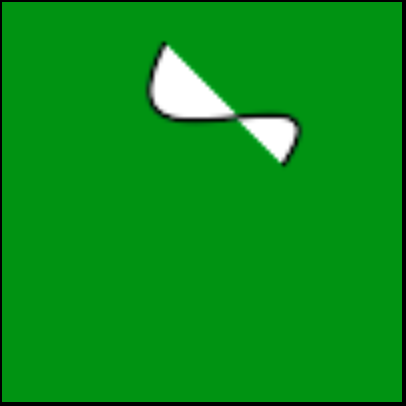# Wand bezier() function in Python

• Last Updated : 11 May, 2020

The bezier() is another Drawing function in Wand. This method is used to draw a bezier curve. It requires four points to determine a bezier curve. Extreme points define the start and end of the curve while in between two points are used to control the curve.

Syntax: wand.drawing.bezier(points)
Parameters :

Attention geek! Strengthen your foundations with the Python Programming Foundation Course and learn the basics.

To begin with, your interview preparations Enhance your Data Structures concepts with the Python DS Course. And to begin with your Machine Learning Journey, join the Machine Learning - Basic Level Course

ParameterInput TypeDescription
pointslistlist of x, y tuples.

Example 1:

 `# Import required objects from wand modules``from` `wand.image ``import` `Image``from` `wand.drawing ``import` `Drawing``from` `wand.color ``import` `Color`` ` `# generate object for wand.drawing``with Drawing() as draw:`` ` `    ``# set stroke color``    ``draw.stroke_color ``=` `Color(``'black'``)`` ` `    ``# set width for stroke``    ``draw.stroke_width ``=` `1`` ` `    ``# points list to determine curve``    ``points ``=` `[(``40``, ``10``), ``# Start point``              ``(``20``, ``50``), ``# First control``              ``(``90``, ``10``), ``# Second control``              ``(``70``, ``40``)] ``# End point `` ` `    ``# fill white color in arc``    ``draw.fill_color ``=` `Color(``'white'``)`` ` `    ``# draw bezier curve using bezier function``    ``draw.bezier(points) ``    ``with Image(width ``=` `100``,``               ``height ``=` `100``,``               ``background ``=` `Color(``'green'``)) as img:`` ` `        ``# draw shape on image using draw() function``        ``draw.draw(img)``        ``img.save(filename ``=``'bezier.png'``)`

Output:Example #2:

Input Image:`# Import required objects from wand modules``from` `wand.image ``import` `Image``from` `wand.drawing ``import` `Drawing``from` `wand.color ``import` `Color`` ` `# generate object for wand.drawing``with Drawing() as draw:``    ``points ``=` `[(``20``, ``100``),  ``# Start point``              ``(``50``, ``10``),  ``# First control``              ``(``50``, ``90``),  ``# Second control``              ``(``180``, ``100``)]`` ` `    ``# set stroke color``    ``draw.stroke_color ``=` `Color(``'black'``)`` ` `    ``# set width for stroke``    ``draw.stroke_width ``=` `1`` ` `    ``# fill white color in arc``    ``draw.fill_color ``=` `Color(``'white'``)`` ` `    ``# draw bezier curve using bezier function``    ``# From bottom left around to top right``    ``draw.bezier(points) ``    ``with Image(filename ``=``"gog.png"``) as img:`` ` `        ``# draw shape on image using draw() function``        ``draw.draw(img)``        ``img.save(filename ``=``'bezier2.png'``)`

Output:My Personal Notes arrow_drop_up